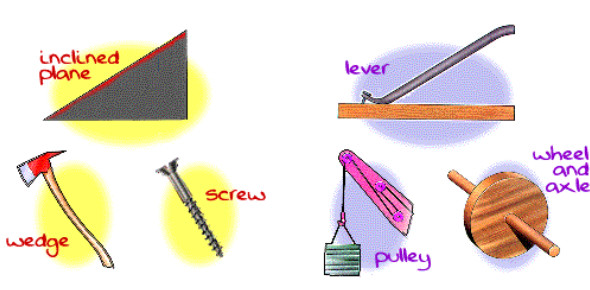# Simple Machines And Tools: Trivia Quiz!

10 Questions | Total Attempts: 3244SettingsSimple machines are devices or tools with few or no mechanical moving parts or tools that make work more comfortable, like a wedge, wheel, axle, lever, pulley, etc. , which are used in construction purposes since time immemorial. This quiz will assess your knowledge about simple machines and tools. Let's try it out!

• 1.
How many classes of levers are there?
• A.

One

• B.

Two

• C.

Three

• D.

Four

• E.

Five

• 2.
A baseball bat is an example of this class of lever:
• A.

First-class

• B.

Second-class

• C.

Third-class

• D.

Fourth-class

• E.

None of the above

• 3.
The formula for the mechanical advantage of an inclined plane is:
• A.

Input arm divided by the output arm

• B.

Distance divided by height

• C.

Height divided by distance

• D.

Output arm divided by input arm

• E.

1/2 input arm divided by output arm

• 4.
All of these are examples of inclined planes except:
• A.

Hatchet

• B.

Ramp

• C.

Staircase

• D.

Slide

• E.

Wheel

• 5.
All of these are types of gears except:
• A.

Snake

• B.

Worm

• C.

Spur

• D.

Bevel

• E.

Rack and pinion

• 6.
These two simple machines make a screw:
• A.

Wheel and axle and inclined plane

• B.

Inclined plane and wedge

• C.

Lever and wedge

• D.

Pulley and lever

• E.

Gear and wedge

• 7.
What simple machine combines with itself to make a wedge?
• A.

Lever

• B.

Inclined plane

• C.

Gear

• D.

Wheel and axle

• E.

Pulley

• 8.
All of these are examples of a wheel and axle except:
• A.

Nail

• B.

Rolling pin

• C.

Roller skates

• D.

Door knob

• E.

Windmill

• 9.
The handles of a pair of scissors are an example of this type of simple machine.
• A.

Gear

• B.

Wheel and axle

• C.

Pulley

• D.

Wedge

• E.

Lever

• 10.
What is a simple machine that uses grooved wheels and a rope to raise, lower, or moves a load?
• A.

Lever

• B.

Wheel and axel

• C.

Inclined plane

• D.

Pulley

Related TopicsBack to top